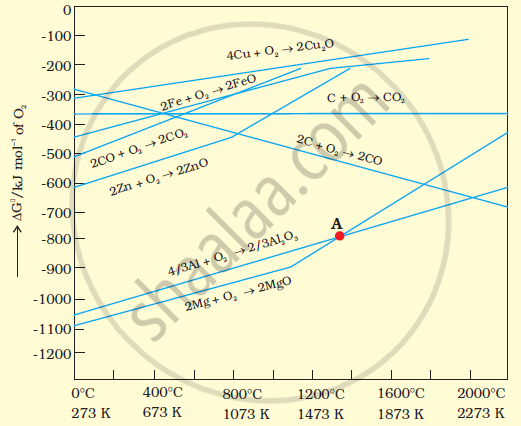PUC Karnataka Science Class 12Department of Pre-University Education, Karnataka
Share

Books Shortlist

The choice of a reducing agent in a particular case depends on thermodynamic factor. How far do you agree with this statement? Support your opinion with two examples. - PUC Karnataka Science Class 12 - Chemistry

ConceptApplication of Thermodynamic Principles of Metallurgy

Question

The choice of a reducing agent in a particular case depends on thermodynamic factor. How far do you agree with this statement? Support your opinion with two examples.

Solution 1The above figure is a plot of Gibbs energy  (triangleG^theta)vs. T for formation of some oxides.

It can be observed from the above graph that a metal can reduce the oxide of other metals, if the standard free energy of formation (triangle_fG^theta) of the oxide of the former is more negative than the latter. For example, since triangle_fG_(Al,Al_2,O_3)^theta is more negative than triangle_fG_(Cu,Cu_2.O)^theta, Al can reduce Cu2O to Cu, but Cu cannot reduce Al2O3. Similarly, Mg can reduce ZnO to Zn, but Zn cannot reduce MgO because triangle_fG_(" " (Mg,MgO))^theta is more negative than triangle_fG_(""   (Zn,ZnO))^(theta).

Solution 2

We can study the choice of a reducing agent in a particular case using Ellingham diagram.

It is evident from the diagram that metals for which the standard free energy of formation oftheir oxides is more negative can reduce those metal oxides for which the standard free energy of formation of their respective oxides is less negative. It means that any metal will reduce the oxides of other metals which lie above it in the Ellingham diagram. This is because the standard free energy change (ΔrG°) of the combined redox reaction will be negative by an amount equal to the difference in Δf G° of the two metal oxides. Thus both Al and Zn can reduce FeO to Fe but Fe cannot reduce Al203 to A1 and ZnO to Zn. In the same way, G can reduce ZnO to Zn but not CO.

Note : Only that reagent will be preferred as reducing agent which will lead to decrease in free energy value (ΔG°) at a certain specific temperature

Solution 3

We can study the choice of a reducing agent in a particular case using Ellingham diagram.

It is evident from the diagram that metals for which the standard free energy of formation oftheir oxides is more negative can reduce those metal oxides for which the standard free energy of formation of their respective oxides is less negative. It means that any metal will reduce the oxides of other metals which lie above it in the Ellingham diagram. This is because the standard free energy change (ΔrG°) of the combined redox reaction will be negative by an amount equal to the difference in Δf G° of the two metal oxides. Thus both Al and Zn can reduce FeO to Fe but Fe cannot reduce Al203 to A1 and ZnO to Zn. In the same way, G can reduce ZnO to Zn but not CO.

Note : Only that reagent will be preferred as reducing agent which will lead to decrease in free energy value (ΔG°) at a certain specific temperature

Is there an error in this question or solution?

APPEARS IN

NCERT Solution for Chemistry Textbook for Class 12 (2015 to Current)
Chapter 6: General Principles and Processes of Isolation of Elements
Q: 23 | Page no. 164
Solution The choice of a reducing agent in a particular case depends on thermodynamic factor. How far do you agree with this statement? Support your opinion with two examples. Concept: Application of Thermodynamic Principles of Metallurgy.
S